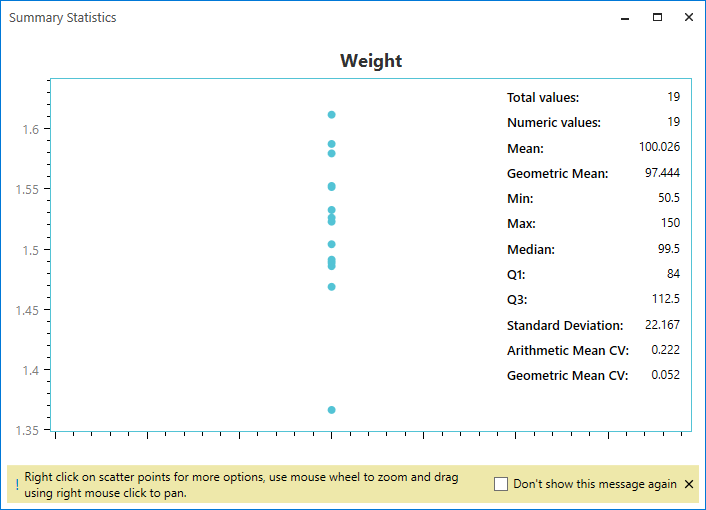Services
Products
News & Events

# Summary Statistics

Statistics can be calculated on specific variables very easily. The mean, median, min, max, standard deviation,geometric mean, Q1, Q3, arithmetic mean CV, and geometric mean CV can be calculated, with the variable values plotted as points on a same vertical axis to easily spot outliers. Pivot tables can also be created to compute statistics grouped by specific variables.Request for proposal